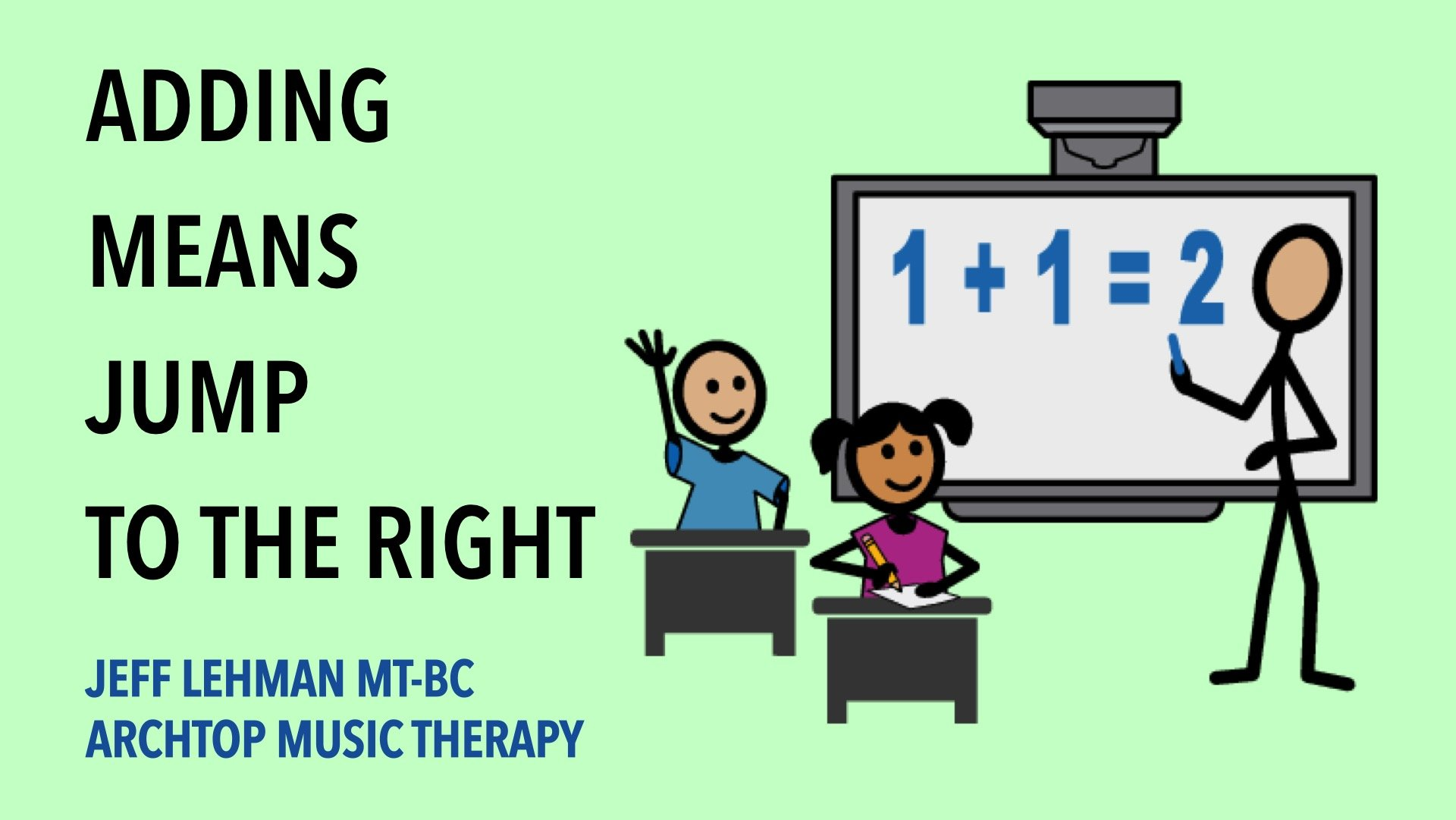Music Therapy math video & song for special needs individuals.
Goal: Clients will solve addition problems using a number line.

Math is hard. Teaching of addition is often done with manipulatives or TouchMath. While these methods can be effective, sometimes it is difficult to tell whether the student genuinely grasps the process of addition or whether they are just demonstrating the skill of counting. Using a number line is an alternative method to aid in understanding and test for comprehension.

These videos present the procedure of adding using a number line: start on the first number of the problem, jump to the right the quantity of times indicated by the second number of the problem, and land on the solution of the problem.

There are eight videos with four math problems each. Four of the videos focus on where to start, with the first number of the problem being the same every time. The other four videos keep the second number of the problem the same to focus on jumping the correct amount of places.

Along with the 8 videos are 8 matching songs for when your student is ready to switch from watching to participating. 5 additional fill-in-the-blank songs (with the math problem vocals removed) are included if you want your student to sing, to move on the number line without verbal prompts, or if you want to switch the order of the math problems or even try different math problems. Full-page math problem visuals corresponding to each of the videos are included, both with and without solutions. Two number lines, a table version and floor mat version, are also included.

• +1 (1+1=2, 2+1=3, 3+1=4, 4+1=5)
• +2 (1+2=3, 2+2=4, 3+2=5, 4+2=6)
• +3 (1+3=4, 2+3=5, 3+3=6, 4+3=7)
• +4 (1+4=5, 2+4=6, 3+4=7, 4+4=8)
• 1+ (1+1=2, 1+2=3, 1+3=4, 1+4=5)
• 2+ (2+1=3, 2+2=4, 2+3=5, 2+4=6)
• 3+ (3+1=4, 3+2=5, 3+3=6, 3+4=7)
• 4+ (4+1=5, 4+2=6, 4+3=7, 4+4=8)
• +1, +2, +3, +4, 1+, 2+, 3+, 4+
• +1 (fill-in-the-blank)
• +2 (fill-in-the-blank)
• +3 (fill-in-the-blank)
• +4 (fill-in-the-blank)
• +1 +2 +3 +4 (fill-in-the-blank)